# `sklearn.gaussian_process`.GaussianProcessRegressor¶

class `sklearn.gaussian_process.``GaussianProcessRegressor`(kernel=None, alpha=1e-10, optimizer='fmin_l_bfgs_b', n_restarts_optimizer=0, normalize_y=False, copy_X_train=True, random_state=None)[source]

Gaussian process regression (GPR).

The implementation is based on Algorithm 2.1 of Gaussian Processes for Machine Learning (GPML) by Rasmussen and Williams.

In addition to standard scikit-learn estimator API, GaussianProcessRegressor:

• allows prediction without prior fitting (based on the GP prior)
• provides an additional method sample_y(X), which evaluates samples drawn from the GPR (prior or posterior) at given inputs
• exposes a method log_marginal_likelihood(theta), which can be used externally for other ways of selecting hyperparameters, e.g., via Markov chain Monte Carlo.

Read more in the User Guide.

New in version 0.18.

Parameters: kernel : kernel object The kernel specifying the covariance function of the GP. If None is passed, the kernel “1.0 * RBF(1.0)” is used as default. Note that the kernel’s hyperparameters are optimized during fitting. alpha : float or array-like, optional (default: 1e-10) Value added to the diagonal of the kernel matrix during fitting. Larger values correspond to increased noise level in the observations and reduce potential numerical issue during fitting. If an array is passed, it must have the same number of entries as the data used for fitting and is used as datapoint-dependent noise level. Note that this is equivalent to adding a WhiteKernel with c=alpha. Allowing to specify the noise level directly as a parameter is mainly for convenience and for consistency with Ridge. optimizer : string or callable, optional (default: “fmin_l_bfgs_b”) Can either be one of the internally supported optimizers for optimizing the kernel’s parameters, specified by a string, or an externally defined optimizer passed as a callable. If a callable is passed, it must have the signature: ```def optimizer(obj_func, initial_theta, bounds): # * 'obj_func' is the objective function to be maximized, which # takes the hyperparameters theta as parameter and an # optional flag eval_gradient, which determines if the # gradient is returned additionally to the function value # * 'initial_theta': the initial value for theta, which can be # used by local optimizers # * 'bounds': the bounds on the values of theta .... # Returned are the best found hyperparameters theta and # the corresponding value of the target function. return theta_opt, func_min ``` Per default, the ‘fmin_l_bfgs_b’ algorithm from scipy.optimize is used. If None is passed, the kernel’s parameters are kept fixed. Available internal optimizers are: ```'fmin_l_bfgs_b' ``` n_restarts_optimizer : int, optional (default: 0) The number of restarts of the optimizer for finding the kernel’s parameters which maximize the log-marginal likelihood. The first run of the optimizer is performed from the kernel’s initial parameters, the remaining ones (if any) from thetas sampled log-uniform randomly from the space of allowed theta-values. If greater than 0, all bounds must be finite. Note that n_restarts_optimizer == 0 implies that one run is performed. normalize_y : boolean, optional (default: False) Whether the target values y are normalized, i.e., the mean of the observed target values become zero. This parameter should be set to True if the target values’ mean is expected to differ considerable from zero. When enabled, the normalization effectively modifies the GP’s prior based on the data, which contradicts the likelihood principle; normalization is thus disabled per default. copy_X_train : bool, optional (default: True) If True, a persistent copy of the training data is stored in the object. Otherwise, just a reference to the training data is stored, which might cause predictions to change if the data is modified externally. random_state : integer or numpy.RandomState, optional The generator used to initialize the centers. If an integer is given, it fixes the seed. Defaults to the global numpy random number generator. X_train_ : array-like, shape = (n_samples, n_features) Feature values in training data (also required for prediction) y_train_ : array-like, shape = (n_samples, [n_output_dims]) Target values in training data (also required for prediction) kernel_ : kernel object The kernel used for prediction. The structure of the kernel is the same as the one passed as parameter but with optimized hyperparameters L_ : array-like, shape = (n_samples, n_samples) Lower-triangular Cholesky decomposition of the kernel in `X_train_` alpha_ : array-like, shape = (n_samples,) Dual coefficients of training data points in kernel space log_marginal_likelihood_value_ : float The log-marginal-likelihood of `self.kernel_.theta`

Methods

 `fit`(X, y) Fit Gaussian process regression model `get_params`([deep]) Get parameters for this estimator. `log_marginal_likelihood`([theta, eval_gradient]) Returns log-marginal likelihood of theta for training data. `predict`(X[, return_std, return_cov]) Predict using the Gaussian process regression model `sample_y`(X[, n_samples, random_state]) Draw samples from Gaussian process and evaluate at X. `score`(X, y[, sample_weight]) Returns the coefficient of determination R^2 of the prediction. `set_params`(\*\*params) Set the parameters of this estimator.
`__init__`(kernel=None, alpha=1e-10, optimizer='fmin_l_bfgs_b', n_restarts_optimizer=0, normalize_y=False, copy_X_train=True, random_state=None)[source]
`fit`(X, y)[source]

Fit Gaussian process regression model

Parameters: X : array-like, shape = (n_samples, n_features) Training data y : array-like, shape = (n_samples, [n_output_dims]) Target values self : returns an instance of self.
`get_params`(deep=True)[source]

Get parameters for this estimator.

Parameters: deep : boolean, optional If True, will return the parameters for this estimator and contained subobjects that are estimators. params : mapping of string to any Parameter names mapped to their values.
`log_marginal_likelihood`(theta=None, eval_gradient=False)[source]

Returns log-marginal likelihood of theta for training data.

Parameters: theta : array-like, shape = (n_kernel_params,) or None Kernel hyperparameters for which the log-marginal likelihood is evaluated. If None, the precomputed log_marginal_likelihood of `self.kernel_.theta` is returned. eval_gradient : bool, default: False If True, the gradient of the log-marginal likelihood with respect to the kernel hyperparameters at position theta is returned additionally. If True, theta must not be None. log_likelihood : float Log-marginal likelihood of theta for training data. log_likelihood_gradient : array, shape = (n_kernel_params,), optional Gradient of the log-marginal likelihood with respect to the kernel hyperparameters at position theta. Only returned when eval_gradient is True.
`predict`(X, return_std=False, return_cov=False)[source]

Predict using the Gaussian process regression model

We can also predict based on an unfitted model by using the GP prior. In addition to the mean of the predictive distribution, also its standard deviation (return_std=True) or covariance (return_cov=True). Note that at most one of the two can be requested.

Parameters: X : array-like, shape = (n_samples, n_features) Query points where the GP is evaluated return_std : bool, default: False If True, the standard-deviation of the predictive distribution at the query points is returned along with the mean. return_cov : bool, default: False If True, the covariance of the joint predictive distribution at the query points is returned along with the mean y_mean : array, shape = (n_samples, [n_output_dims]) Mean of predictive distribution a query points y_std : array, shape = (n_samples,), optional Standard deviation of predictive distribution at query points. Only returned when return_std is True. y_cov : array, shape = (n_samples, n_samples), optional Covariance of joint predictive distribution a query points. Only returned when return_cov is True.
`sample_y`(X, n_samples=1, random_state=0)[source]

Draw samples from Gaussian process and evaluate at X.

Parameters: X : array-like, shape = (n_samples_X, n_features) Query points where the GP samples are evaluated n_samples : int, default: 1 The number of samples drawn from the Gaussian process random_state: RandomState or an int seed (0 by default) : A random number generator instance y_samples : array, shape = (n_samples_X, [n_output_dims], n_samples) Values of n_samples samples drawn from Gaussian process and evaluated at query points.
`score`(X, y, sample_weight=None)[source]

Returns the coefficient of determination R^2 of the prediction.

The coefficient R^2 is defined as (1 - u/v), where u is the regression sum of squares ((y_true - y_pred) ** 2).sum() and v is the residual sum of squares ((y_true - y_true.mean()) ** 2).sum(). Best possible score is 1.0 and it can be negative (because the model can be arbitrarily worse). A constant model that always predicts the expected value of y, disregarding the input features, would get a R^2 score of 0.0.

Parameters: X : array-like, shape = (n_samples, n_features) Test samples. y : array-like, shape = (n_samples) or (n_samples, n_outputs) True values for X. sample_weight : array-like, shape = [n_samples], optional Sample weights. score : float R^2 of self.predict(X) wrt. y.
`set_params`(**params)[source]

Set the parameters of this estimator.

The method works on simple estimators as well as on nested objects (such as pipelines). The latter have parameters of the form `<component>__<parameter>` so that it’s possible to update each component of a nested object.

Returns: self :

## Examples using `sklearn.gaussian_process.GaussianProcessRegressor`¶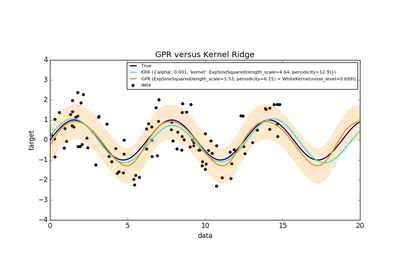Comparison of kernel ridge and Gaussian process regression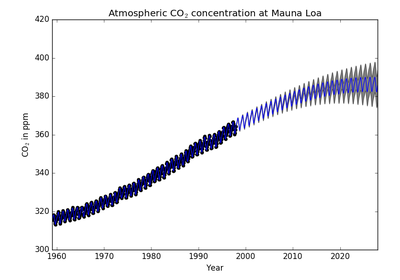Gaussian process regression (GPR) on Mauna Loa CO2 data.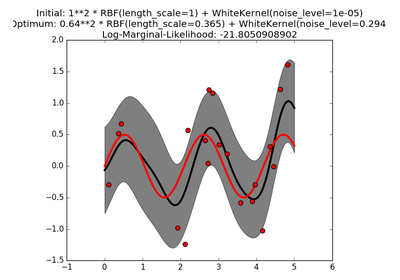Gaussian process regression (GPR) with noise-level estimation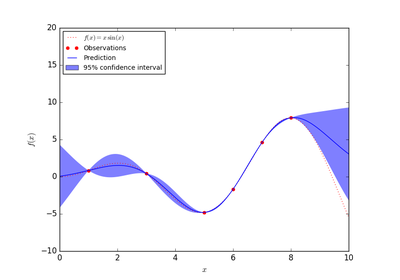Gaussian Processes regression: basic introductory example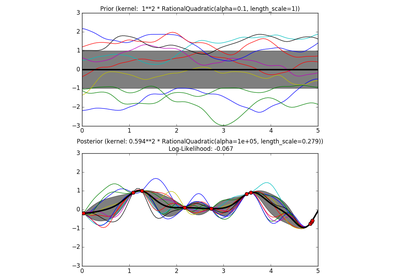Illustration of prior and posterior Gaussian process for different kernels